De Claron Ltd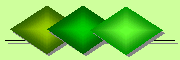Express Accountancy services at affordable prices
 Accounting Property Investment Reporting Company Secretarial Services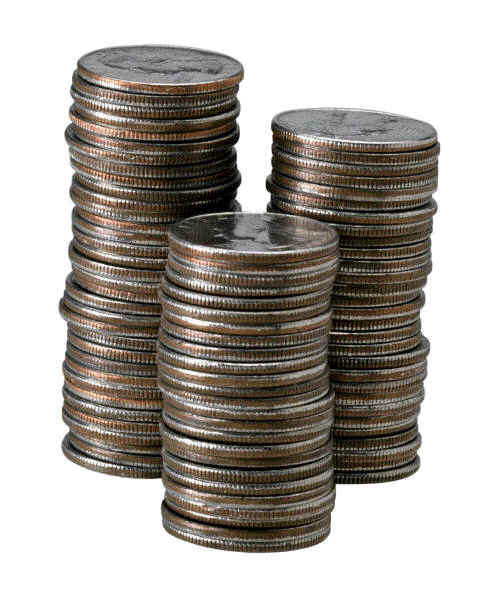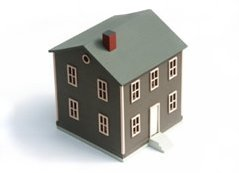Help

Cost of Capital

The cost of capital is the weighted cost of the debt and equity of associated with a property. It is used to evaluate the profitability of investing in a new property on the basis of the minimum return that the property is exepected to generate for the investor. For an investment property to be worthwhile, the expected return on capital must be greater than the cost of capital.

Cost of Debt
The cost of debt is any interest paid on a mortgage for acquiring an investment property. This is adjusted for tax-deductions claimed for interest as a business expense. Within this context fluctuations in the cost of debt can occur due to changes to:

• the size of the mortgage
• interest rates
• tax rates
• Note that fluctuations in the size and duration of the mortgage can be directly influenced by the lender who can decide:

• on the size of the mortgage taken
• to pay off the the mortgage sooner
• whether mortgage repayments are solely interest based or a mix of interest and capital repayments
• The cost of debt should also take into consideration a risk premium. This premium takes into account any anticipated rises in interest rates, adverse fluctations in tax rates and any penalties associated with the inability to pay off the mortgage as per schedule.

Given the above, the formula for cost of debt can be expressed as:

• The after-tax cost of debt on capital = interest rate + risk premium on long-term debt x (1 - the marginal tax rate as %)
• For example, a company acquires an investment property with an interest only non-deposit mortgage of \$100,000 which attracts an annual interest rate of 4.2%. The risk premium is estimated at 1.4% and the corporation tax rate is 21%. Using the above formula the annual cost of debt is 4.42% which equates to \$4,424.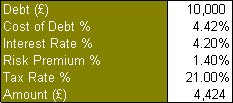Cost of Equity
The cost of equity is the implicit opportunity cost of foregoing an alternative investment. This can be calculated using Capital Asset Pricing Model" (CAPM). In relation to property investments this model says that an investor will demand a minimum rate of return equal to the return from a risk-free investment plus a return for bearing extra risk. This extra risk is called the "equity risk premium", and is equivalent to the risk premium of the market as a whole times a multiplier called "beta" that measures how risky a specific investment property is relative to the total market. The formula for the cost of equity is:

• Cost of Equity = Risk-Free Rate + (Beta x Market Risk Premium).
• The above formula can be illustrated by a property that is bought for \$100,000. If this same amount of money was invested in government bonds that gave a guaranteed yield of 5% per annum then this is the risk-free rate.

However, investment properties are not risk free. Such properties are expected to generate a return in terms of rent or sales. However, these expected returns carry risks as rent or sales maybe lower than expected or do not occur within projected time scales. This is the market risk premium of investment properties and in the above example is estimated at 4%.

There is the additional consideration of the relative volatility or stability of rent or sales. This determines the Beta value. The Beta value is the additional amount of return expected by the property investor to compensate for any volatility in rent or sales. The higher volatility then the greater the Beta value. In the above example the Beta value is estimated at 1.0.

Using the above formula the cost of equity for the property bought for \$100,000 is 9% or \$9,000.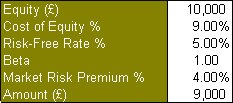Weighted Cost of Capital
The weighted cost of capital is the respective contributions of the cost of debt and the cost of equity to total capital cost. In the above example, the cost of debt is \$4,424 and the cost of equity is \$9,000 giving a total capital cost of \$13,424. By proportion the cost of debt comprises 33% of this total and the cost of equity comprises the remaining 67%. Together these comprise the weighted cost of capital for the above investment property acquired at \$100,000.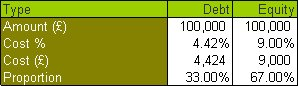Related topics:

• Capital Asset Pricing Model" (CAPM)
• Discounted Cash Flows